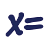Games
Problems
Go Pro!

# Beginner Programming Tips

Reference > Science > TechnologyToggling a variable between two values in a computer program; switching a bit from zero to one and back.Random number generators -- creating and understanding how they work, with Basic example using Rnd function and int function.Does an equation have a value? Yes, you can find the values of equations - True or False, in Boolean terms.Counting truth values -- how many equations in a list are true or false? Counting up the true statements.Testing for zero -- three methods to determine if one or more computer variables is equal to zero.Walk through an array -- convert a two-dimensional index to a one-dimensional index. Use a function for reusable code.Walk through an array -- convert a one-dimensional index to a two-dimensional index, using integer division and modulus math.Removing Array Elements -- how to remove an element from an array, using basic programming techniques.Parsing a string containing a comma-separated list of values to extract the values and perform some action with those values.Given a number of seconds, convert that into minutes or hours. Uses the Mod function, Integer division, and the Format function.A function to make words either plural or singular, depending on whether an integer variable is one or not.Every time you select a section of code to copy and paste, ask yourself, 'Should this be a function?'Randomizing an array: how to you scramble the order of elements in an array?Randomizing an array by randomizing a second array containing indices into the first array.Use the random function to generate random passwords.How to validate an e-mail address entered by a site visitor. Does the e-mail address have the proper format?Like us on Facebook to get updates about new resources